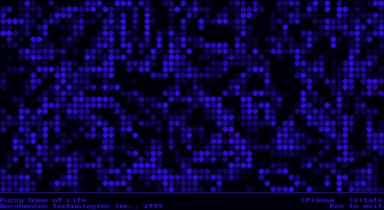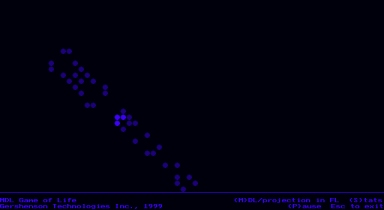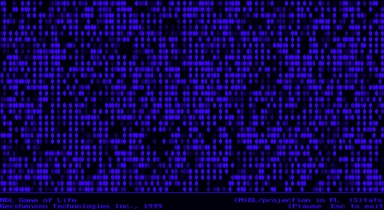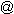Fuzzy Game of Life

Here you can find a Game of Life with fuzzy rules:

-Every cell has values between 0 and 1.

-If the sum of the values of the 8 cells around x (let-s call it C) <= 1 or >= 4, and x>.5 then x=0.

-If 1<C<2 and x>.5 then x=C-1

-If 2<=C<=3 and x>.5 then x=1

-If 3<C<4 and x>.5 then x=4-C

(these last 4 ifs are the equivalents for the ifs of a cell with value 1. The next 2 would be the equivalent for a cell with value of 0)

-If x<.5 and 2<C<=3 then x=C-2

-If x<.5 and 3<C<4 then x=4-C

The original figures (gliders, traffic lights, spaceships, etc.) also work here...

To draw it, we draw a scale from black to blue to show 0 to 1.The program was implemented in Borland C 3.1 for DOS. Yes, I know it's a crappy interface, but it´s also an easy one, and I haven't understood VRML and Java completely. You can download this program, source and some example files here.

MDL Game of Life

Well, having the fuzzy game of life, I just changed the fuzzy rules into multidimensional ones.

So instead of having every cell a value between 0 and 1, it has 2: one for it's life and one for it's death.The other rules are very similar to the fuzzy game of life.

Very strange and interesting stuff happens here. Check it out!You can choose between seeing the projection of bidimensional logic in fuzzy logic (above), or seeing both values (life, death) at the same time (below)This also was implemented in crappy old Borland C 3.1 for DOS, and you can downoad the program, source and examples here.

Any comments are welcome, and please tell me if you find any interesting properties while running these games of life. Althrought they are chaotic, they are very stable in the proportions they keep of cells alive & dead (the chaos is constant?).

E-mail: cggunam.mxMultidimensional Logic resources at: http://turing.iimas.unam.mx/%7Ecgg/jlagunez/mdl